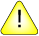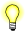This version of this document is no longer maintained. For the latest documentation, see http://www.qnx.com/developers/docs.

# nextafter(), nextafterf()

Compute the next representable double-precision floating-point number

## Synopsis:

```#include <math.h>

double nextafter ( double x,
double y);

float nextafterf ( float x,
float y );```

## Arguments:

x
The number that you want the next number after.
y
A number that specifies the direction you want to go; see below.

## Library:

libm

Use the -l m option to qcc to link against this library.

## Description:

The nextafter() and nextafterf() functions compute the next representable double-precision floating-point value following x in the direction of y.

## Returns:

The next machine floating-point number of x in the direction towards y.

If: nextafter() returns:
y < x The next possible floating-point value less than y
y > x The next possible floating-point value greater than x
x is NAN NAN
y is NAN NAN
x is finite +/-HUGE_VAL, according to the sign of x (errno is set)If an error occurs, these functions return 0, but this is also a valid mathematical result. If you want to check for errors, set errno to 0, call the function, and then check errno again. These functions don't change errno if no errors occurred.

## Examples:

```#include <stdio.h>
#include <errno.h>
#include <inttypes.h>
#include <math.h>
#include <fpstatus.h>

void dump_to_hex(double d) {
printf("0x%08x %08x \n",
(uint32_t)(*((uint64_t*)&d) >> 32),
(uint32_t)(*((uint64_t*)&d)));
}

int main(int argc, char** argv)
{
double a, b, c;

a = 0 ;
b = nextafter(a, -1);
c = nextafter(a, 1);
printf("Next possible value before %f is %f \n", a, b);
printf("-->"); dump_to_hex(a);
printf("-->"); dump_to_hex(b);
printf("Next possible value after %f is %f \n", a, c);
printf("-->"); dump_to_hex(a);
printf("-->"); dump_to_hex(c);

return(0);
}```

produces the output:

```Next possible value before 0.000000 is 0.000000
-->0x00000000 00000000
-->0x80000000 00000001
Next possible value after 0.000000 is 0.000000
-->0x00000000 00000000```

## Classification:

Safety:
Cancellation point No
Interrupt handler No
Signal handler No